A closer look at Functions

The following topics are covered in this section:

Recursion

Recursion comes from the word ‘to recur’ which means happening repeatedly over and over again. Recursion is also referred to as circular definition.

In programming we have recursive functions and they are functions that keep repeating themselves. How do they repeat themselves? Well, actually the function keeps calling itself till some condition is satisfied. It might seem really funny to think of a function calling itself but this is exactly what happens. The best example for recursion is to calculate factorials. In the last program we wrote a function to find the factorial of a number using the ‘for’ loop. We’ll now see how to do the same using recursion. Remember to read the program just like the compiler would read it; otherwise you’re bound to get confused.

#include <iostream.h>
int fact(int n)
{
int result;
if (n= =1)
{
return 1;
}
else
{
result = n * fact(n-1);
return result;
}
}

int main( )
{
int num;
cout<<"\nFor what function do you want to find the factorial : ";
cin>>num;
cout<<"\n\n The result is : "<<fact(num);
return 0;
}

First of all, the function fact ( ) is the recursive function here. Why? Check out the function body and you’ll see that this function calls itself. The basic idea is that if you want to find the factorial of 3, the answer is actually equal to 3*factorial (2). The factorial of 2 will be: 2*factorial (1) and factorial of 1 will be 1.

Let us interpret the logic of the program. For understanding purpose, let’s assume that we want to find the factorial of 4.

• Now num=4 and n=4. The compiler enters into the recursive function and calculates value of ‘result’.
• result = 4* fact (3);

what does fact(3) mean? ‘fact’ is the same function and hence the function is being called with a different argument value. The value returned by the function is ‘result’ (which is 4*fact(3)). But there is another function called in this returned value. So the program tries to calculate fact(3). When calculating fact(3), it again calculates the value of ‘result’. Now,

• result=3*fact(2);

This value is returned and your actual expression in the computer would be 4*3*fact(2). What next?

• Calculate fact(2) and this leads to:

result=2*fact(1);

• The expression in the computer will now be 4*3*2*fact(1). The program calculates fact(1). When the value of ‘n’ is 1, we have specified an ‘if’ condition that says: return 1;
• 1 is returned for fact (1) and the entire expression becomes: 4*3*2*1 and the compiler produces the result by multiplying the numbers.

If you don’t give the ‘if’ condition the recursive nature will never stop and it will lead to problems.

Remember: When using recursive functions make sure that there is a way for the function to stop itself from infinitely repeating itself.

Can it repeat itself infinitely? You do not want a function to keep calling itself infinitely and this is why we should provide some means of breaking out from recursion. When using recursion there are chances for stack overflow. Stack is a special portion of memory where the computer stores temporary values. For example when a computer encounters a function call, it has to store the current location before going to the function. When the function is executed, the program will return back to the original position and continue the program execution. In recursive functions, there are chances for the stack getting used up quickly. That’s what is stack overflow.

Anyway, there isn’t much advantage of using recursion but there may be some cases where you feel that recursion might make the coding easier. A programmer usually uses recursion only if it mirrors the natural methodology or if the iteration method is complex. But be very careful while using recursion. The problem with recursion is that it involves a series of function calls and this can consume extra memory space.

Remember: Any C++ function can be made recursive. What about main( )? It is also a C++ function but main ( ) is the one function which CANNOT be made recursive.

Recursion in string reversal

It is important to understand how the stack works during recursion. The best example to illustrate this is the problem of reversing a given string. The problem would be easy to solve if we knew the length of the string beforehand. Or we could declare a huge array and hope that we don’t get a string longer than the array. Our problem is stated as follows:

"Write a C++ program to reverse a string entered by the user. The string will be terminated when the user presses the Enter key. You should not restrict the input string length."

To solve this let’s take a recursive approach:

//*********************************************************
//* Program to illustrate string reversal using recursion                                  *
//*********************************************************

#include <iostream>
using namespace std;

void backwards( )
{
char ch;
ch=getchar( );
if (ch!='\n')
{
backwards( );
}
cout<<ch;
}

int main( )
{
backwards( );
return 0;
}

The output would be:

trial

lairt

Though the code is really small it might seem confusing at first. First of all, the getchar( ) function will extract only one character at a time. If you want to retrieve 5 characters then you’d have to use getchar( ) 5 times (in our program we do this using recursion). To explain the working, let’s take an example of what happens when we run the program.

The main( ) function calls the function backward( ). In this we have a local variable named ‘ch’. This is now allocated memory space in the stack. Let’s consider our stack as shown below (‘ch’ still doesn’t have a value):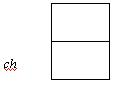The next line says:

ch = getchar( );

On encountering this line the program waits till the user presses an Enter (getchar( ) will retrieve a character only when the user presses the Enter key). The user now types the word ‘trial’ and presses Enter.

‘ch’ will now have the value ‘t’ (1st letter of the input string).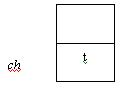Next line says:

if (ch!='\n')
{
backwards( );
}

Our ‘ch’ value is ‘t’. So the condition is true and backwards( ) is called again. What happens in this second call?

Remember: When a function call occurs the compiler stores some information in memory so that it can return back to the caller. If function A calls B, then once B is completed the program flow should return to the exact point in function A and continue executing the rest of function A.

In our case the backward function is not complete (it will be complete when backward( ) returns control to the caller- in our case the caller is main( ) ). So, again we go through the same process. A character named ‘ch’ is declared in the function. Thus memory space is allocated for this variable in the stack.

Remember: Each time a local function is called the variables created within that function are available only within that function.

So now our stack will look as below: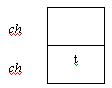Why is the second ‘ch’ on top of the first? A stack functions like that. Each time you add something new onto the stack, it will be added to the top of the pile (just like a stack of plates). The first ‘ch’ and second ‘ch’ are not the same. The top ‘ch’ corresponds to the variable created during the second call of backward( ) while the lower one corresponds to the variable created in the first call of backward( ). Let’s denote them as ch1 and ch2 (so that you don’t get confused as to which ch we are referring to).

Again we have a getchar( ) function. This will now get the second letter of the input string (i.e. ‘r’). Now ‘ch’ has a value of ‘r’ and it is not equal to \n. The stack would like this: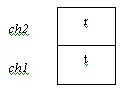Again the function backward( ) is called. This process goes on and you will finally reach the following stack structure: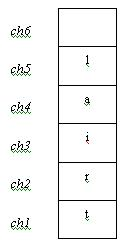This ‘ch’ will have a value of ‘\n’. The if condition will fail and we’ll enter the else loop which says:

cout<<ch;

Since ‘ch’ is ‘\n’ the program will output a newline character onto the screen. What happens next?

We still have 5 functions that have not executed their entire block of code (before each backward( ) could complete we called another backward( )- so now the program has to keep going backwards). After printing the newline character on the screen, the current backward( ) function ends (it reaches the terminating } ). So now control goes back to its caller. The caller was the 5th execution of the function backwards( ). This has a line of code that says:

cout<<ch;

In this case, ‘ch’ refers to its local variable (this ‘ch’ was pushed onto the stack – we refer to it as ch5 in our illustration). So this will cause the program to print ‘l’ on the screen. This function ends here and control returns to the caller of this function and so on. Thus you get the string in reverse order.

Remember: Once a function terminates (i.e. returns control to the caller, the local variables used by that function are popped out of the stack- i.e. they are lost once the function returns control to the caller).

Inline Functions

There may be instances when you want to repeat a process more than once in a program. You may think of going for the concept of functions. It’s fine as long as your function is a bit lengthy. Suppose there are only one or two lines you want to repeat? Is it worthwhile going for a function?

When a program executes a function call, it has to save the present memory address and then go to a new memory address to access the function. After executing the function it has to return back to the memory address from where it branched off. All of this is referred to as ‘overhead’ and by branching (or jumping) from the main program to sub-routines involves a lot of overhead. This means it will take some time and will also use some memory space.

If a function is very small, then it might not be advisable to make use of so much of overhead (i.e. there is no need for using another function).

Instead, we can make use of the inline functions. Inline functions will simply substitute the function definition wherever there is a function call. This is more like substitution.

Example:

// Convert Dollars to Rupees
# include <iostream.h>
inline double dollartors (double d)             // The function is inline.
{
return 47*d;                                 // Inline functions usually contain only one/two body lines
}

int main ( )
{
double dollar;
cout << "How many dollars :";
cin>>dollar;
cout<< "Rupees : "<<dollartors(dollar);             //Function call
return 0;
}

As you can see above, the inline function is very similar to a normal function. One difference is that you have to mention the keyword ‘inline’ before the function name in the declaration. When the compiler comes to the function call, it knows that ‘dollartors’ is an inline function. So the compiler will insert the body of the function in place of the function call. The compiler will simply copy and paste the function body wherever there is a function call. After making the substitutions wherever the inline function has been called (in our program it is done in only one place), the program is executed.

Thus when the program is executed, the amount of overhead involved is reduced since the equivalent coding will be:

int main ( )
{
double dollar;
cout << "How many dollars :";
cin>>dollar;
cout<< "Rupees : "<< 47*d; //Inline function body substituted
return 0;
}

The advantage is that the program needn’t save its present memory address, go to the function’s memory address, execute the function and return back to the original address. Instead the function code is brought into the main program and it is executed just like a normal C++ statement. Thus processing time can be reduced significantly.

Remember: It is useful to make a function inline if its body consists of just one or two lines.

Recap

• A function is a group of statements written for a specific purpose and grouped within a single block.
• A function has to be declared if it is called before being defined.
• Arguments are passed to a function’s parameters.
• The data type of the arguments should match the data type of the parameters.
• If a function is declared as returning a value to the caller then it should return the corresponding data type value (unless it returns void).
• The return statement is used to return a value to the caller as well as give back program control to the caller.
• The main ( ) function returns value to the OS.
• Overloaded functions should have the same name but different parameters.
• Return data type cannot be used as a basis for function overloading.
• Inline functions are used when the body of the function is very small (one or two lines).
• Recursive functions are functions that call themselves. Some provision has to be provided for them to break out of recursivity.
• Recursive functions have more overheads and are generally not used.

Go back to the Contents Page